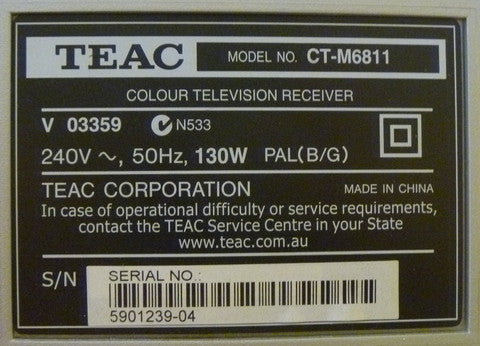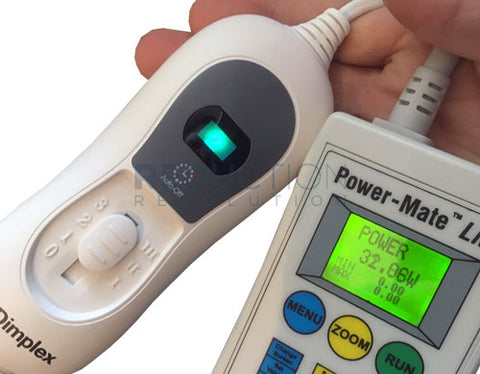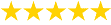# How to Calculate Watts (Wattage) - Mains Power

The wattage of an appliance (in watts) is often said to be current (in amps) multiplied by voltage (in volts).

Whilst this is true for simplified or direct current (DC) circuits, it's not the case for the mains power we use every day.

This conventional wisdom or 'rule of thumb' will have you calculate Apparent Power rather than Real Power.

### How NOT to Calculate Wattage - Apparent Power (VA)

Amps (A) x Volts (V) = Volt-Amps (VA)

The formula above can be used for calculating apparent power consumption in volt-amperes (VA). This equation will give you a rough idea of power use in watts but is not strictly correct. For this, you need to take into account the power factor.

## How to Calculate Wattage - Real Power (Watts)

Amps (A) x Volts (V) x Power Factor = Watts (W)

This formula takes the power factor into account and shows accurate power consumption (what you are billed for).

### What is Power Factor?

Power Factor is a measure of the effectiveness with which an electrical device converts volt-amperes into watts. Power factor is represented as a dimensionless number between 0 and 1.

The closer the number is to one, the 'better' the power factor. The higher the power factor, the more effectively electrical power is used. Resistive loads, such as most electric heaters, will have a power factor of 1 as they convert all electrical power supplied into heat. Equipment with motors, such as fridges and air conditioners, will have a lower power factor.

## How Does This Relate to Watts & Wattage?

Power factor is crucial if you want to know the actual power consumption of an appliance. See below for a demonstration of how power factor is used with our power meter to calculate the real energy consumption of a small TV.

Larger businesses need to have a power factor close to 'unity' (1) as they may be charged a fee if they have a low power factor. This is because the utility has to supply more current (amps) to the site than required. In so doing, they incur more transmission losses. The good news is that businesses can take steps to increase their power factor.

## Example - Calculate the Actual Watts Of A TV

The compliance label on this TV shows the power use as 130 Watts.The problem is that compliance labels often show maximum power rather than actual power. The only way to know the real power is to measure it with a plug-in power meter. Over a two hours, the power meter showed a power draw of between 70 and 110 Watts - substantially less than indicated on the label.A watt meter like this will calculate the actual wattage.

At one point the power meter showed the TV to be using 243 volts and 0.421 Amps. If we follow conventional wisdom and just multiply Volts and Amps together without power factor, we'd work out the apparent power draw as follows:-

• Amps (A) X Volts (V) = VA
• 243 V x 0.421 A = 102.3 VA

...then falsely present it as 102.3 W

When we add power factor into the calculation, we get a very different figure. Since the power meter showed a power factor of 0.65 at that time, the calculation becomes:

• Amps (A) x Volts (V) x Power Factor = Watts (W)
• 234 V x 0.421 A x 0.65 = 66.5 W

Hopefully, you can now see why it's essential to get this calculation right.

Thankfully, our plug-in power meters will do these calculations for you. Our power meter displays real power (Watts) as well as Amps (A), Volts (V), and power factor so you can verify the calculation if you need to.The Reduction Revolution Plug-in Power Meter is our cheapest and most popular option. The Power Mate Lite (pictured above) is a high accuracy model used by professional energy auditors.

|

### Got a Question? We're Here to Help!Read Our Customer Reviews!